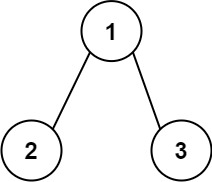# Solved! Leetcode 129. Sum Root to Leaf Numbers

### Sum Root to Leaf Numbers

You are given the root of a binary tree containing digits from 0 to 9 only.
Each root-to-leaf path in the tree represents a number.
For example, the root-to-leaf path 1 -> 2 -> 3 represents the number 123.
Return the total sum of all root-to-leaf numbers. Test cases are generated so that the answer will fit in a 32-bit integer.

A leaf node is a node with no children.

Example 1:Input: root = [1,2,3]
Output: 25
Explanation:

• The root-to-leaf path 1->2 represents the number 12.
• The root-to-leaf path 1->3 represents the number 13.
Therefore, sum = 12 + 13 = 25.

Example 2:Input: root = [4,9,0,5,1]
Output: 1026
Explanation:

• The root-to-leaf path 4->9->5 represents the number 495.
• The root-to-leaf path 4->9->1 represents the number 491.
• The root-to-leaf path 4->0 represents the number 40.
Therefore, sum = 495 + 491 + 40 = 1026.

Constraints:

• The number of nodes in the tree is in the range [1, 1000].
• 0 <= Node.val <= 9
• The depth of the tree will not exceed 10.

n: total number of nodes in a tree
Time Complexity:

• O(n): since we need to visit each node in a Tree

Space complexity:

• O(1)
Rate this post